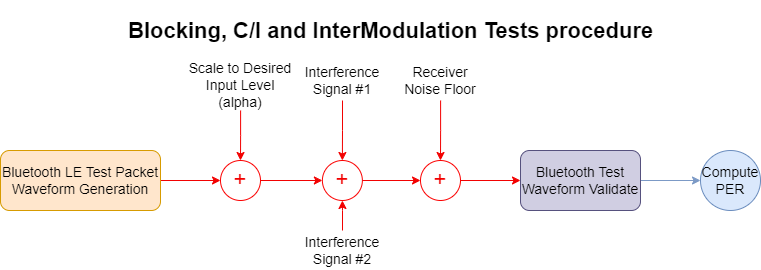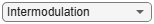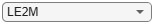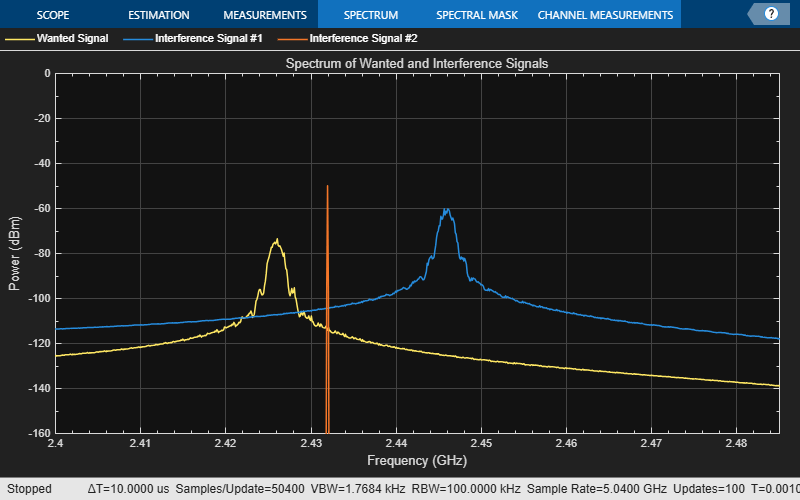BLE Blocking, Intermodulation and Carrier to Interference Performance Tests

This example shows how to model Bluetooth® low energy (BLE) RF-PHY receiver tests specific to blocking, intermodulation and carrier to interference (C/I) performance as per the Bluetooth RF-PHY Test Specifications [ 1 ] using Communications Toolbox™ Library for the Bluetooth Protocol.

Background

The Bluetooth RF-PHY Test Specifications [ 1 ] defined by Bluetooth special interest group (SIG) includes RF-PHY tests for both transmitter and receiver. The objectives of these RF-PHY tests are to ensure interoperability between all BLE devices and to verify that a basic level of system performance is guaranteed for all BLE products. Each test case has a specified test procedure and an expected outcome, which must be met by the implementation under test (IUT).

Introduction

The Bluetooth receiver tests are designed to ensure that the IUT can receive data over a range of conditions where the transmitted signal has high power, and in presence of both in-band and out-of-band interference with a defined packet error rate (PER). This example covers three BLE RF-PHY receiver tests for blocking, intermodulation and C/I performance as per the Bluetooth RF-PHY Test Specifications [ 1 ].

• Blocking Performance: The blocking performance test verifies the receiver performance in the presence of out-of-band interfering signals i.e. operating outside the 2400 MHz - 2483.5 MHz band.

• Intermodulation Performance: The intermodulation performance test verifies the receiver performance in presence of unwanted signals nearby in frequency.

• C/I Performance: The C/I performance test verifies the receiver performance in presence of adjacent and co-channel interfering signals.

All the above RF-PHY tests are necessary because the wanted signal often will not be the only signal transmitting in the given frequency range.

The following block diagram summarizes the example flow.1. Generate test packets and pass through bleWaveformGenerator to generate BLE test waveform.

2. Perform frequency upconversion to obtain a passband signal.

3. Scale the transmitted signal to a desired input level.

4. Add the interference signal(s) depending on the performance test.

6. At the receiver, down convert the signal and then demodulate, decode and perform CRC check.

7. Measure the PER based on CRC check and then compare it with the reference PER.

Check for Support Package Installation

% Check if the 'Communications Toolbox Library for the Bluetooth Protocol'
% support package is installed or not.
commSupportPackageCheck('BLUETOOTH');

Initialize the Simulation Parameters

You can change rxPerformanceTest, phyMode and Fc parameters based on the receiver performance test, PHY transmission mode and frequency of operation, respectively.

rxPerformanceTest ='Intermodulation'; % Select one from the set {'C/I', 'Blocking', 'Intermodulation'}
% Select PHY transmission mode as per Bluetooth RF-PHY Test Specifications
phyMode ='LE1M'; % {LE1M, LE2M, LE500K, LE125K} for C/I
% {LE1M, LE2M} for blocking and intermodulation
% Select frequency of operation for IUT based on the performance test and
% generic access profile (GAP) role(s) as shown in the table below.
% --------------------------------------------------------------------------------
% Operating | Peripheral & Central Devices    | Broadcaster & Observer Devices   |
% Frequency |                                 |                                  |
% (MHz)     |---------------------------------|----------------------------------|
%           | C/I  | Blocking |Intermodulation| C/I  | Blocking | Intermodulation|
% ----------|------|----------|---------------|------|----------|----------------|
%   Lowest  | 2406 |    -     |    2402       | 2402 |    -     |    2402        |
%   Middle  | 2440 |    2426  |    2440       | 2426 |    2426  |    2426        |
%   Highest | 2476 |    -     |    2480       | 2480 |    -     |    2480        |
% --------------------------------------------------------------------------------
Fc = 2426e6; % Frequency of operation in Hz
payloadLength =37; % Payload length in bytes, must be in the range [37,255]
sps = 40; % Number of samples per symbol

% Calculate sampling rate in Hz based on PHY transmission mode
Rsym = 1e6;
if strcmp(phyMode,'LE2M')
Rsym = 2e6;
end
Fs = Rsym*sps;

Generate Baseband Waveforms

The function, helperBLETestWaveform.m, can be configured to generate a BLE test packet waveform as per the Bluetooth specifications [ 2 ]. In this example, wanted and interference baseband waveforms can be generated by changing the payload type parameter.

% Generate a wanted signal which is always a modulated carrier with a PRBS9

% Generate an interference signal #1 which is a modulated carrier with a

Frequency Upconversion

Apply frequency upconversion to obtain a passband signal for the specified frequency of operation.

% Interpolation factor for upconversion to cover BLE RF frequency band
% (2400e6 to 2485e6)
interpFactor = ceil(2*2485e6/Fs);

% Create a digital upconverter System object
upConv = dsp.DigitalUpConverter(...
'InterpolationFactor',interpFactor,...
'SampleRate',Fs,...
'Bandwidth',2e6,...
'StopbandAttenuation',44,...
'PassbandRipple',0.5,...
'CenterFrequency',Fc);

% Upconvert the baseband waveform to passband
wantedWaveformUp = upConv([wantedWaveform;zeros(8*sps,1)]);

Generate Test Parameters

Test parameters are generated based on performance test, frequency of operation and PHY transmission mode. The function, helperBLETestParamGenerate.m, is used to generate all the interference frequencies and corresponding scaling factors (alpha, beta, gamma) for selected receiver performance test.

[alpha,beta,gamma,interferenceFreq1,interferenceFreq2] = ...
helperBLETestParamGenerate(rxPerformanceTest,Fc,phyMode);

Repeat test parameters based on the number of packets used for simulation.

pktCnt = 10; % Number of packets
maxInterferenceParams = min(length(interferenceFreq1),pktCnt); % Maximum number of interference parameters used for simulation

% Repeat all the interference parameters such that PER can be averaged over
% the entire range of interference frequencies for selected receiver
% performance test.
repFact = ceil(pktCnt/maxInterferenceParams); % Repetition factor
betaRep = repmat(beta,repFact,1);
gammaRep = repmat(gamma,repFact,1);
interferenceFreq1Rep = repmat(interferenceFreq1,repFact,1);
interferenceFreq2Rep = repmat(interferenceFreq2,repFact,1);

Test Simulation

In this example, all the three BLE RF-PHY performance tests are simulated as follows:

• For Blocking performance, there will be only one interference signal i.e. interference signal #2. So, the scaling factor (beta) for interference signal #1 is zero.

• For Intermodulation performance, there will be two interference signals.

• For C/I performance, there will be only one interference signal i.e. interference signal #1. So, the scaling factor (gamma) for interference signal #2 is zero.

% Upconvert and store the interference waveform #1 based on buffer
% size, so that the stored interference waveforms can be reused if
% the packet count exceeds the buffer size.
interferenceWaveform1Up = zeros(length(wantedWaveformUp),maxInterferenceParams);
if any(strcmp(rxPerformanceTest,{'C/I','Intermodulation'}))
for i=1:maxInterferenceParams
release(upConv)
upConv.CenterFrequency = interferenceFreq1Rep(i);
interferenceWaveform1Up(:,i) = upConv([interferenceWaveform1;zeros(8*sps,1)]);
end
end

% Initialize a variable for reusing the interference waveform #1
j = rem(1:pktCnt,maxInterferenceParams);
j(j == 0) = maxInterferenceParams;

% Create a digital down converter System object
downConv = dsp.DigitalDownConverter(...
'DecimationFactor',interpFactor,...
'SampleRate',Fs*interpFactor,...
'Bandwidth',2e6,...
'StopbandAttenuation',44,...
'PassbandRipple',0.5,...
'CenterFrequency',Fc);

% Create automatic gain control System object
agc = comm.AGC('DesiredOutputPower',1);

% Create a thermal noise System object
NF = 12; % Noise figure (dB)
thNoise = comm.ThermalNoise('NoiseMethod','Noise figure',...
'SampleRate',interpFactor*Fs,...
'NoiseFigure',NF);

% Time vector to generate sinusoidal unmodulated interference signal i.e.
% interference signal #2.
t = (0:(length(wantedWaveformUp)-1)).'/(interpFactor*Fs);
pktLost = 0; % Initialize counter
for i=1:pktCnt

% Generate an interference waveform #2 which is a sinusoidal
% unmodulated signal. The sqrt(2) factor ensures that the power of the
% sinusoidal signal is normalized.
interferenceWaveform2 = sqrt(2)*sin(2*pi*interferenceFreq2Rep(i)*t);

% Add the interference signals to wanted signal
rxWaveform = alpha*wantedWaveformUp + betaRep(i)*interferenceWaveform1Up(:,j(i)) + gammaRep(i)*interferenceWaveform2;
chanOut = thNoise(complex(rxWaveform)); % Add thermal noise to the signal
downConvOut = downConv(real(chanOut)); % Perform frequency down conversion
agcOut = agc(downConvOut); % Apply AGC
'SamplesPerSymbol',sps,'WhitenStatus','Off'); % Extract message information
pktLost = pktLost + crcFail;
end

% Determine the PER
per = pktLost/pktCnt;

Spectrum Visualization

Create and configure a spectrum analyzer and show the spectrum of last transmitted wanted signal and interference signal(s) based on the receiver performance test.

% Setup spectrum viewer
spectrumScope = dsp.SpectrumAnalyzer( ...
'SampleRate',            interpFactor*Fs,...
'SpectralAverages',      10,...
'YLimits',               [-160 0], ...
'Title',                 'Spectrum of Wanted and Interference Signals',...
'SpectrumUnits',         'dBm',...
'NumInputPorts' ,        2,...
'ChannelNames',          {'Wanted Signal','Interference Signal'},...
'ShowLegend',            true,...
'FrequencySpan',         'Start and stop frequencies',...
'StartFrequency',        2400e6,...
'StopFrequency',         2485e6,...
'RBWSource',             'Property',...
'RBW',                   1e5,...
'PlotAsTwoSidedSpectrum',false);

if strcmp(rxPerformanceTest,'C/I')
spectrumScope(alpha*wantedWaveformUp,betaRep(end)*interferenceWaveform1Up(:,end))
elseif strcmp(rxPerformanceTest,'Blocking')
spectrumScope.StartFrequency = 30e6;
spectrumScope(alpha*wantedWaveformUp,gammaRep(end)*interferenceWaveform2)
else
spectrumScope.NumInputPorts = 3;
spectrumScope.ChannelNames = {'Wanted Signal','Interference Signal #1','Interference Signal #2'};
spectrumScope(alpha*wantedWaveformUp,betaRep(end)*interferenceWaveform1Up(:,end),gammaRep(end)*interferenceWaveform2)
endReference Results

This section generates the reference PER values for each PHY transmission mode based on the payload length as specified in section 6.4 of the Bluetooth RF-PHY Test Specifications [ 1 ].

berTable = [0.1 0.064 0.034 0.017]*0.01;
refBER = berTable(1);
refBER = berTable(2);
refBER = berTable(3);
else
refBER = berTable(4);
end
crcLengthBytes = 3; % CRC length in bytes
fprintf('Measured PER and reference PER for payload length of %d bytes are %f, %f respectively.\n',payloadLength,per,refPER);
Measured PER and reference PER for payload length of 37 bytes are 0.000000, 0.308010 respectively.
if per <= refPER
fprintf('%s performance test passed.\n',rxPerformanceTest);
else
fprintf('%s performance test failed.\n',rxPerformanceTest);
end
Intermodulation performance test passed.

Appendix

This example uses the following helper functions:

Selected Bibliography

1. Bluetooth RF-PHY Test Specification.

2. Volume 6 of the Bluetooth Core Specification, Version 5.0 Core System Package [Low Energy Controller Volume].